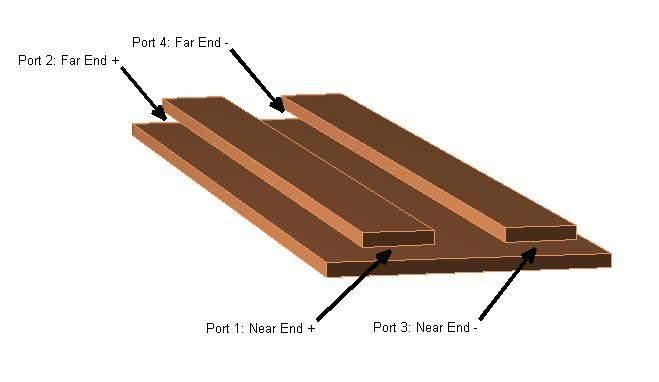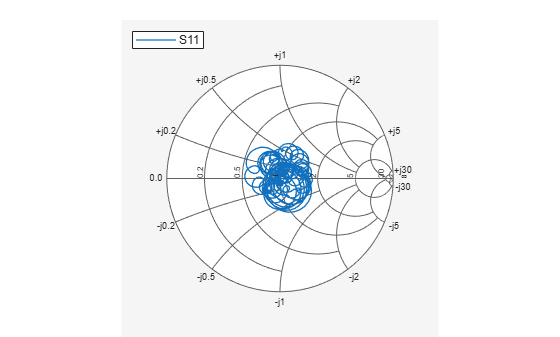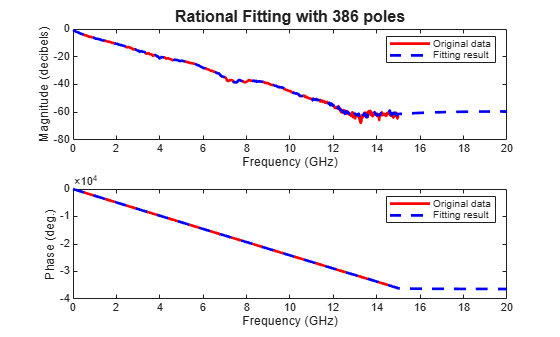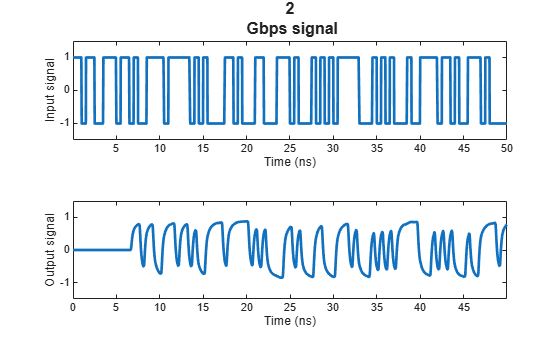Modeling a High-Speed Backplane (4-Port S-Parameters to a Rational Function)

This example shows how to use RF Toolbox™ to model a differential high-speed backplane channel using rational functions. This type of model is useful to signal integrity engineers, whose goal is to reliably connect high-speed semiconductor devices with, for example, multi-Gbps serial data streams across backplanes and printed circuit boards.

Compared to traditional techniques such as linear interpolation, rational function fitting provides more insight into the physical characteristics of a high-speed backplane. It provides a means, called model order reduction, of making a trade-off between complexity and accuracy. For a given accuracy, rational functions are less complex than other types of models such as FIR filters generated by IFFT techniques. In addition, rational function models inherently constrain the phase to be zero on extrapolation to DC. Less physically-based methods require elaborate constraint algorithms in order to force the extrapolated phase to zero at DC.Figure 1: A differential high-speed backplane channel

Read the Single-Ended 4-Port S-Parameters and Convert Them to Differential 2-Port S-Parameters

Read a Touchstone® data file, default.s4p, into an sparameters object. The parameters in this data file are the 50-ohm S-parameters of the single-ended 4-port passive circuit shown in Figure 1, given at 1496 frequencies ranging from 50 MHz to 15 GHz. Then, get the single-ended 4-port S-parameters and use the matrix conversion function s2sdd to convert them to differential 2-port S-parameters. Finally, plot the differential S11 parameter on a Smith chart.

filename = 'default.s4p';
backplane = sparameters(filename);
data = backplane.Parameters;
freq = backplane.Frequencies;
z0 = backplane.Impedance;

Convert to 2-port differential S-parameters.

diffdata = s2sdd(data);
diffz0 = 2*z0;

By default, s2sdd expects ports 1 & 3 to be inputs and ports 2 & 4 to be outputs. However if your data has ports 1 & 2 as inputs and ports 3 & 4 as outputs, then use 2 as the second input argument to s2sdd function to specify this alternate port arrangement. For example, diffdata = s2sdd(data,2);

diffsparams = sparameters(diffdata,freq,diffz0)
diffsparams =
sparameters: S-parameters object

NumPorts: 2
Frequencies: [1496x1 double]
Parameters: [2x2x1496 double]
Impedance: 100

rfparam(obj,i,j) returns S-parameter Sij

figure
smithplot(diffsparams,1,1)Compute the Transfer Function and Its Rational Function Object Representation

First, use the s2tf function to compute the differential transfer function. Then, use the rationalfit function to compute the analytical form of the transfer function and store it in an rfmodel.rational object. The rationalfit function fits a rational function object to the specified data over the specified frequencies. The run time depends on the computer, the fitting tolerance, the number of data points, etc.

difftransfunc = s2tf(diffdata,diffz0,diffz0,diffz0);
delayfactor = 0.98; % Delay factor. Leave at the default of zero if your
% data does not have a well-defined principle delay
rationalfunc = rationalfit(freq,difftransfunc,'DelayFactor',delayfactor)
rationalfunc =
rfmodel.rational with properties:

A: [31x1 double]
C: [31x1 double]
D: 0
Delay: 6.5521e-09
Name: 'Rational Function'

npoles = length(rationalfunc.A);
fprintf('The derived rational function contains %d poles.\n',npoles);
The derived rational function contains 31 poles.

Validate the Differential-Mode Frequency Response

Use the freqresp method of the rfmodel.rational object to get the frequency response of the rational function object. Then, create a plot to compare the frequency response of the rational function object and that of the original data. Note that detrended phase (i.e. phase after the principle delay is removed) is plotted in both cases.

freqsforresp = linspace(0,20e9,2000)';
resp = freqresp(rationalfunc,freqsforresp);

figure
subplot(2,1,1)
plot(freq*1.e-9,20*log10(abs(difftransfunc)),'r',freqsforresp*1.e-9, ...
20*log10(abs(resp)),'b--','LineWidth',2)
title(sprintf('Rational Fitting with %d poles',npoles),'FontSize',12)
ylabel('Magnitude (decibels)')
xlabel('Frequency (GHz)')
legend('Original data','Fitting result')

subplot(2,1,2)
origangle = unwrap(angle(difftransfunc))*180/pi+360*freq*rationalfunc.Delay;
plotangle = unwrap(angle(resp))*180/pi+360*freqsforresp*rationalfunc.Delay;
plot(freq*1.e-9,origangle,'r',freqsforresp*1.e-9,plotangle,'b--', ...
'LineWidth',2)
ylabel('Detrended phase (deg.)')
xlabel('Frequency (GHz)')
legend('Original data','Fitting result')Calculate and Plot the Differential Input and Output Signals of the High-Speed Backplane

Generate a random 2 Gbps pulse signal. Then, use the timeresp method of the rfmodel.rational object to compute the response of the rational function object to the random pulse. Finally, plot the input and output signals of the rational function model that represents the differential circuit.

datarate = 2*1e9;                % Data rate: 2 Gbps
samplespersymb = 100;
pulsewidth = 1/datarate;
ts = pulsewidth/samplespersymb;
numsamples = 2^17;
numplotpoints = 10000;

t_in = double((1:numsamples)')*ts;
input = sign(randn(1,ceil(numsamples/samplespersymb)));
input = repmat(input,[samplespersymb, 1]);
input = input(:);
[output,t_out] = timeresp(rationalfunc,input,ts);

figure
subplot(2,1,1)
plot(t_in(1:numplotpoints)*1e9,input(1:numplotpoints),'LineWidth',2)
title([num2str(datarate*1e-9),' Gbps signal'],'FontSize',12)
ylabel('Input signal')
xlabel('Time (ns)')
axis([-inf,inf,-1.5,1.5])

subplot(2,1,2)
plot(t_out(1:numplotpoints)*1e9,output(1:numplotpoints),'LineWidth',2)
ylabel('Output signal')
xlabel('Time (ns)')
axis([-inf,inf,-1.5,1.5])Plot the Eye Diagram of the 2-Gbps Output Signal

Estimate and remove the delay from the output signal and create an eye diagram by using Communications Toolbox™ functions.

if ~isempty(which('eyediagram'))
ignoreBits = 1500;
eyediagram(output(ignoreBits:end),2*samplespersymb,2/datarate)
end